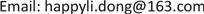1海军装备部驻沈阳地区第二军事代表室，辽宁 沈阳

291899部队，辽宁 葫芦岛

392728部队，上海

4海军航空大学，山东 烟台1. 引言

2. 备件供应工作内容2.1. 备件目录种类

1) 随机备件目录：主要以在飞机的保证期内，用于恢复飞机主机、机载成品与地面保障设备的效能所需的不可修复件和部分可修复件为主要内容。不可修复件包括消耗性备件和易损性备件。可修备件主要指飞机维修性所划定的一些外场可更换件。

2) 推荐订货备件目录：国外亦称为后续备件目录，是由承包商向用户推荐的订货备件目录，以用于保证期后(包括部分保证期内的不属于随机备件范围内的备件)恢复系统效能和保证飞机持续使用所需的备件为主要内容。其由使用方订货，费用不计入飞机成本。

2.2. 备件供应体制

3. 备件需求量计算方法3.1. 备件需求量计算模型

{ min ∑ i = 1 n c i x i , i = 1 , 2 , ⋯ , n ∏ i = 1 n R i ( x i , t i ) > R 0 (1)

R i = P { N i ( t ) ≤ x i } (3)

R s = P { N 1 ( t ) + N 2 ( t ) + ⋯ + N n ( t ) ≤ ( x 1 + x 2 + ⋯ + x n ) } (4)

x i 0 = { x i , N i ( t ) ≥ x i N i ( t ) , N i ( t ) < x i (5)

R ^ s = ∑ i = 1 n x i 0 ∑ i = 1 n N i ( t ) = ∑ i = 1 n N i ( t ) ∑ i = 1 n N i ( t ) R i (6)

R s = ∑ i = 1 n λ i ∑ i = 1 n λ i R i (7)

ρ i = λ i ∑ i = 1 n λ i , i = 1 , 2 , ⋯ , n ，

R s = ∑ i = 1 n ρ i R i (8)

1) 基于一定经费的后续备件保障模型的目标函数为

R = max ∑ i = 1 N ρ ( i ) R ( i ) (9)

C = ∑ i = 1 N C ( i ) X ( i ) ≤ C ( 0 ) (10)

C ( i ) 为第i个备件的单价，式10表示对各部件的数量和单价的乘积进行求和并且使其值小于或等于系统总经费 C ( 0 ) 。

R ( 00 ) ≤ R ( X ( i ) , T ) , i = 1 , 2 , ⋯ , k , R ( 01 ) ≤ R ( 00 ) ≤ R ( 02 ) (12)

3.2. 基于保障率的后续备件保障模型

C = min ∑ i = 1 N C ( i ) X ( i ) (13)

R ( 01 ) ≤ R ( X ( i ) , T ) ≤ R ( 02 ) (14)

∑ i = 1 N ρ ( i ) R ( i ) ≥ R ( 0 ) (15)

∑ i = 1 J − 1 ρ ( i ) R ( i ) + ∑ i = J + 1 N ρ ( i ) R ( i ) + ρ ( J ) × R ( X ( J ) − 1 , T ) ≤ R ( 0 ) (16)

J为 1 , ⋯ , N 中的任一数值。表示当第J个备件缺一个时系统总保障率R小于 R ( 0 ) 。

3.3. 优化保障模型

1) 构造判断矩阵

a i j = 1 a j i , a i i = 1 , ( i , j = 1 , 2 , ⋯ , n ) (17)

a i j = [ a i j − , a i j + ] = { [ a i j − δ , a i j + δ ] ( a i j ≥ 1 , i ≠ j ) [ 1 1 / a i j + δ , 1 1 / a i j − δ ] ( a i j ≤ 1 , i ≠ j ) [ 1 , 1 ] ( i = j ) (18)

a i j 为模糊判断矩阵中的元素，因此判断矩阵 A ( k ) 为正互反矩阵。

2) 计算归一化权重向量

α = [ ∑ j = 1 n 1 ∑ i = 1 n a i j + ] 1 / 2 , β = [ ∑ j = 1 n 1 ∑ i = 1 n a i j − ] 1 / 2 (19)

C . I . = ( α λ max − + β λ max + ) / 2 − n n − 1 (20)

3) 计算决策权重

w = 1 ∑ k = 1 k y k ∑ k = 1 k y k w 0 ( k ) (21)

4) 部件的最终权重

4. 某型发动机备件方案分析4.1. 备件权重

Importance compariso

A ( 1 ) = [ [ 1 , 1 ] [ 1 6 , 1 4 ] [ 1 4 , 1 2 ] [ 4.5 , 5.5 ] [ 4 , 6 ] [ 1 , 1 ] [ 1 6 , 1 4 ] [ 1.5 , 4.5 ] [ 2 , 4 ] [ 4 , 6 ] [ 1 , 1 ] [ 4 , 6 ] [ 1 5.5 , 1 4.5 ] [ 1 4.5 , 1 1.5 ] [ 1 6 , 1 4 ] [ 1 , 1 ] ]

A ( 2 ) = [ [ 1 , 1 ] [ 1 8.5 , 1 5.5 ] [ 1 4 , 1 2 ] [ 4 , 6 ] [ 5.5 , 8.5 ] [ 1 , 1 ] [ 1 4 , 1 2 ] [ 4 , 6 ] [ 2 , 4 ] [ 2 , 4 ] [ 1 , 1 ] [ 5.5 , 8.5 ] [ 1 6 , 1 4 ] [ 1 6 , 1 4 ] [ 1 8.5 , 1 5.5 ] [ 1 , 1 ] ]

x − ( 1 ) = ( 0 .2424,0 .2172,0 .2172,0 .1018 )

x + ( 1 ) = ( 0 .2363,0 .2738,0 .2738,0 .0767 )

4.2. 优化备件保障方案

Weight of main component

15.3253.811.211.76580.098543
212.7835.301.301.69750.094721.5
33.2734.311.211.89370.105612
44.4194.611.512.04930.1143213
56.2254.801.811.91650.106932.9
63.3844.511.411.99750.111424
74.2834.811.601.91140.106618
82.2473.901.901.49710.083515.6
93.3844.801.311.78500.099522
102.2473.801.701.41900.079128

5. 总结